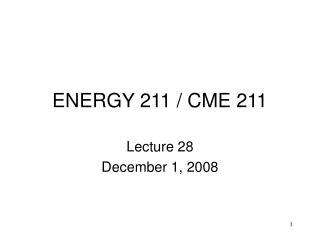DownloadDownload PresentationENERGY 211 / CME 211

ENERGY 211 / CME 211

Download PresentationENERGY 211 / CME 211

- - - - - - - - - - - - - - - - - - - - - - - - - - - E N D - - - - - - - - - - - - - - - - - - - - - - - - - - -
Presentation Transcript

1. ENERGY 211 / CME 211 Lecture 28 December 1, 2008

2. Calling C/C++ from MATLAB • A MEX-file is a C or FORTRAN program that can be called from MATLAB • It is a dynamic-link library that implements a single MATLAB function • Name of library (minus extension) is name of MATLAB function • Most useful to allow existing compiled-language applications to be used from within MATLAB without having to rewrite code

3. MATLAB Arrays • A MATLAB variable is stored in a mxArray • An mxArray contains info about a variable's type, size, etc. and its data • Internally, matrices are stored in column-major order, like in FORTRAN • For complex data, real and imaginary parts are stored in separate arrays • Strings are stored as arrays of unsigned integers, not characters

4. The Interface • The code for your MEX-file must include a function called mexFunction, which is called by MATLAB • It takes these arguments: • int nlhs: number of output arguments • mxArray *plhs[]: array with nlhs elements that point to output arguments • intnrhs: number of input arguments • const mxArray*prhs[]: array with nrhs elements that point to input arguments

5. Working with Arguments • mxGetPr returns a pointer to the real part of an input or output argument • If argument is complex, use mxGetPi to get pointer to imaginary part • mxGetM, mxGetN return the size of a matrix passed as an argument • mxGetScalar returns the value of a scalar argument, as a double • mxCreateDoubleMatrix allocates a matrix for an output argument

6. Building MEX-files in MDS • Project: "Win32 Dynamic-Link Library" • Change Project Settings: • Compile tab: add include directory from MATLAB installation where mex.h is found • Link tab: change Output file name extension from .dll to .mexw32; add mx and mex to list of Libraries; add location of MATLAB's .dll under Additional Library Path • Place library in directory from which it will be run in MATLAB

7. Calling MATLAB from C/C++ • Must #include"engine.h" and link with eng library • engOpen function starts MATLAB • engEvalString evaluates MATLAB command in string s • engGetVariable gets variable from MATLAB workspace and stores mxArray • engPutVariable puts mxArray in MATLAB workspace with given name

8. Next Time • Calling FORTRAN from C/C++ code • And vice versa • Using command-line arguments